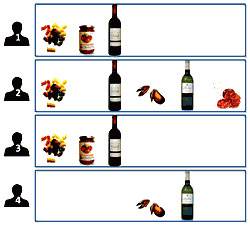ML Wiki

Mining Association Rules

Association Rules

Association rule mining:

• Finding all the rules $X \to Y$ such that
• $P(X \land Y) \geqslant \text{min_supp}$ and
• $P(Y | X) \geqslant \text{min_con}$
• these are predictive patterns

E.g.

• $\text{wings} \to \text{beak}$
• $\text{wings} \land \text{beak} \to \text{fly}$

Association rules

• $X \to Y$ is an association rule if
• $X$ and $Y$ are itemsets
• $X \cap Y = \varnothing$
• $X$ is called the body
• $Y$ is called the head (conclusion)

Motivation

Consider this example

• we data with customers and their purchases
•pasta t.souse red wine seafood white wine salami
1
2
3
4
5

Pattern 1: How to organize supermarket?

• we see that seafood and white wine usually go together, so put them together
• these are associations that are not always observed in practice

Pattern 2: What to promote?

• we see a rule $\text{pasta} \land \text{souse} \to \text{red wine}$
• it doesn't always hold, but it's a typical pattern
• so promote red wine

Generation of Association Rules

Algorithm

Generate(frequent itemsets $F$, confidence threshold $\theta$)

• $R \leftarrow \varnothing$
• for each $X \in F$, for each $Y \subset X$
• if $\text{conf}(Y \subset X) = \cfrac{\text{supp}(X)}{\text{supp}(Y)} \geqslant \theta$
• then $R \leftarrow R \cup \{ Y \to X - Y \}$
• return $R$

Complexity

It computes association rules in polynomial time

Examples

Example 1

${A, B, C, D, E, F}$

• $T_1 = \{A,B,D,E\}$
• $T_2 = \{A,B,C,D,F\}$
• $T_3 = \{B,D,F\}$
• $T_4 = \{C,E,F\}$

• Find rules $X \to A$ ($A$ = Apple) with support threshold 50%
• Calculate the confidence of these rules
• Are there redundant rules?

Frequent items with support 50%:

• $[A, B, C, D, E, F, DF, BF, AD, BD, AB, CF, BDF, ABD]$ - calculated with Apriori
• ones that involve $A$: $[A, AD, AB, ABD]$
• so, rules are $\varnothing \to A, B \to A, D \to A, BD \to A$

Confidence:

• $\text{conf}(\varnothing \to A) = \cfrac{\text{supp}(\varnothing \to A)}{\text{supp}(\varnothing)} = \cfrac{2}{4}$
• $\text{conf}(B \to A) = \cfrac{\text{supp}(B \to A)}{\text{supp}(B)} = \cfrac{2}{3}$
• $\text{conf}(D \to A) = \cfrac{\text{supp}(D \to A)}{\text{supp}(D)} = \cfrac{2}{3}$
• $\text{conf}(BD \to A) = \cfrac{\text{supp}(BD \to A)}{\text{supp}(BD)} = \cfrac{2}{3}$

Redundant rules

• the rule $BD \to A$ is redundant because we have $B \to A$ and $D \to A$